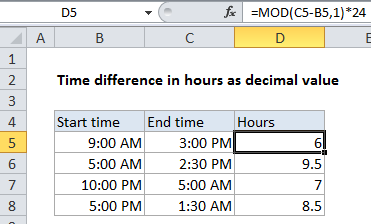## Excel Office

Excel How Tos, Tutorials, Tips & Tricks, Shortcuts

# Calculate time difference in hours as decimal value in Excel

To get the duration between two times in as decimal hour (i.e. 3 hrs, 4.5 hrs, 8 hrs, etc.) you can use a formula based on the MOD function.

## Formula

`=MOD(end-start,1)*24`## Explanation

In the example shown, the formula in D5 is:

`=MOD(B2-A2,1)*24`

### Excel hours

In Excel, one day is the number 1, so 1 hour = 1/24 = 0.041666667. In other words, hours are just fractional parts of a day:

Worked Example:   Count cells that contain odd numbers in Excel
Time Fraction Hours
3:00 AM 0.125 3
6:00 AM 0.25 6
9:00 AM 0.375 9
12:00 PM 0.5 12
3:00 PM 0.625 15
6:00 PM 0.75 18
9:00 PM 0.875 21
12:00 AM 1 24

To convert these fractional values to decimal hours, just multiply by 24. For example .5 * 24 = 12 hours, .24 * 24 = 6 hours, etc.

### Times that cross midnight

When times cross midnight, the problem becomes more tricky, since the end time will often be less than the start time.  One elegant way to handle this challenge is to add the MOD function to the formula. For example, to calculate hours between 9 PM and 3 AM:

```=MOD(0.125-0.875,1)*24
=MOD(-0.75,1)*24
=0.25*24
=6```

The MOD function takes care of the negative problem by “flipping” negative values to the required positive value. (In this way, the MOD function works a bit like a clock.

Worked Example:   Highlight multiples of specific value in Excel

### Hours between times

To calculate hours between times, you can simply subtract the start time from the end time when both times are in the same day. For example, with start time of 9:00 AM and an end time of 3:00 PM, you can simply use this formula:

```=(3:00 PM-9:00 AM)*24
=(.625-.375)*24
=.25 * 24
=6```
Worked Example:   Highlight every other row in Excel